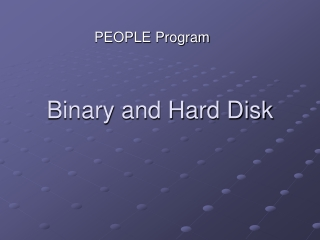DownloadDownload PresentationBinary and Hard Disk

# Binary and Hard Disk

Download Presentation## Binary and Hard Disk

- - - - - - - - - - - - - - - - - - - - - - - - - - - E N D - - - - - - - - - - - - - - - - - - - - - - - - - - -
##### Presentation Transcript

1. PEOPLE Program Binary and Hard Disk

2. How do Computers Store Numbers • Computers are constructed of digital electronics => two states: “on” “off” • Binary number system consists of 0 and 1 only • On-off patterns are used to encode numbers using binary number system

3. Binary numbers are great! • Simple to work with • No big addition and multiplication tables to learn • Just do same things over and over very fast • Just use two values of voltage, magnetism or other signal • Hardware easier to design and more resistant

4. Hard Drive • Hard disks are used in all desktop computers, servers, super computers etc. They are also VCR type devices or video recorders that use hard drives instead of tape • They store changing digital information in a relatively permanent form. They give computers the ability to remember things when the power goes out.

5. How Does Binary Work? • Decimal number system • 10 digits (0 to 9) • Add a second column worth 10 times the value of the first column • Expanded notation: • 3 x 100 + 6 x 10 + 5 = 365 • 1 x 1000 + 0 x 100 + 3 x 10 + 2 = 1032

6. Binary Number System • Only contains two digits: 0,1 • Add a second column worth 2 times the value of the first column • To convert a number from binary to decimal, use expanded notation: • 101101 = 1x32 + 0x16 + 1x8 + 1x4 + 0x2 + 1x1 = 45

7. Binary  Decimal • 1  a power of 2 • 0  zero • Examples • 0001  2^0 = 1 • 0010  2^1 = 2 • 0100  2^2 = 4 • 1000  2^3 = 8 • Any desired amount can be represented using 1 and 0. • Examples • 1 == 0001 • 3 == 0011 • 6 == 0110 • 0101 = 0 + 4 + 0 + 1 = 5 • 1010 = 8 + 0 + 2 + 0 = 10 • 0111 = 0 + 4 + 2 + 1 = 7

8. Larger Numbers • Numbers from 1 to 15 • 0000 = 0 0100 = 4 1000 = 8 1100 = 120001 = 1 0101 = 5 1001 = 9 1101 = 130010 = 2 0110 = 6 1010 = 10 1110 = 140011 = 3 0111 = 7 1011 = 11 1111 = 15 • Bigger whole numbers  more bits more places in binary number • 10000101 = 128 + 0 + 0 + 0 + 0 + 4 + 0 + 1 = 133 • This is 8 bits == 1 byte

9. Larger Numbers • 10000101 = 128 + 0 + 0 + 0 + 0 + 4 + 1 = 133 • This is 8 bits == 1 byte • Kilobyte = 1024 bytes (1024 = 2^10) • Megabyte = 1024 kilobytes ~ a million bytes • Gigabyte = 1024 megabytes

10. Typical Sizes • Typical Hard disks are 100 – 500 gigabyte • 1 byte == 1 character  hard disk might hold 100 billion characters ~ 20 billion words of raw text • Real numbers, fractions, very large numbers  floating point arithmetic

11. Binary Addition • Decimal System • Sum >= 10  add 1 to the column on the left • Binary System • Sum >= 2  add 1 to the column on the left • Example: 1+1+1 = 11 1+1+1+1 = 100 110101 + 11110 -------------- 1010011

12. Binary Addition • Second Way • Convert the numbers to decimal • Add the decimal numbers • Convert the sum to binary

13. ASCII Table • ASCII: American Standard Code for Information Interchange • Alphanumeric characters are represented with 8 bits • A  65 == 01000001 • Write 3 letters in ASCII Table